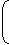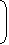# Aptitude - Surds and Indices - Discussion

### Discussion :: Surds and Indices - General Questions (Q.No.12)

12.

 1 + 1 = ? 1 + a(n - m) 1 + a(m - n)

[A]. 0
[B].
 1 2
[C]. 1
[D]. am + n

Explanation:

1 + 1 =
1  +  11 + anam1 + aman
1 + a(n - m) 1 + a(m - n)

 = am + an (am + an) (am + an)

 = (am + an) (am + an)

= 1.

 Sunny Kumar said: (Jul 15, 2014) Could anybody please help me with this as where both 1's in numerator gone? Thanks.

 Karthik said: (Jan 8, 2017) Please solve this sum in details with DIRECTION TO SIMPLIFY EACH VALUES.

 Rianshi Sharma said: (Mar 18, 2017) Step 1. Take negative power as denominator of positive power. Step 2. LCM the whole thing. Step 3. You will get the Result.

 Nischal said: (Aug 22, 2017) Please explain the last step.

 Mohit said: (Aug 30, 2017) The answer should been 1/2.

 Ragav said: (Oct 14, 2018) Here, assign a=1.